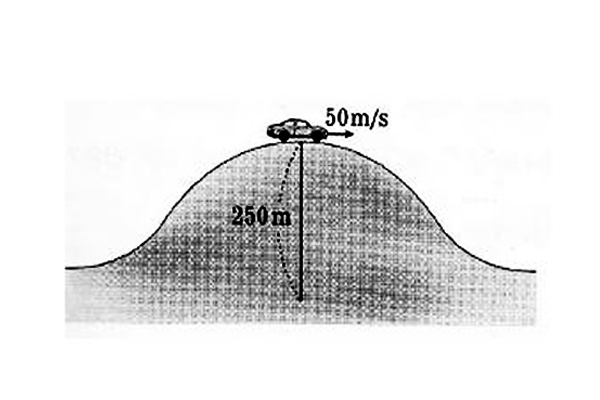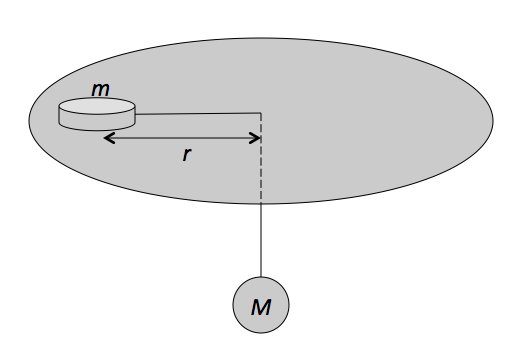Classical Mechanics

# Centripetal acceleration

A rock tied to a string is moving at a constant speed of $8.0 \text{ m/s}$ in a circle of radius $7.0 \text{ m}.$ Calculate the approximate magnitude of the centripetal acceleration of the rock.The above picture is a $1000$ kg car passing the top of a hill with radius of curvature $250$ m at a speed of $50$ m/s. Find the magnitude of normal force on the car (in N).

Gravitational acceleration is $g= 10$ m/s$^{2}$.A puck of mass $m = 1.80 \text{ kg}$ slides in a circle of radius $r = 50.00 \text{ cm}$ on a frictionless table while attached to a hanging ball of mass $M = 3.50 \text{ kg}$ by a cord through a hole in the table, as shown in the figure above. Approximately how fast must the puck move in order to keep the ball at rest?

The gravitational acceleration is $g =10 \text{ m/s}^2.$

A block is hung by a string from the roof of a van. The van drives along a straight, flat road at a constant speed of $15 \sqrt{2} \text{ m/s},$ and the block hangs vertically down. Then the van goes around an unbanked curve of radius $45.0 \text{ m}$ while maintaining the same speed, and the string tilts by an angle of $\theta .$ Find the value of $\theta.$

The gravitational acceleration is $g= 10 \text{ m/s}^2.$

A rock tied to a string is moving at a constant speed of $1.8 \text{ m/s}$ in a circle. If the rock's acceleration is $3.6 \text{ m/s}^2,$ what is the approximate radius of the circle it moves in?

×